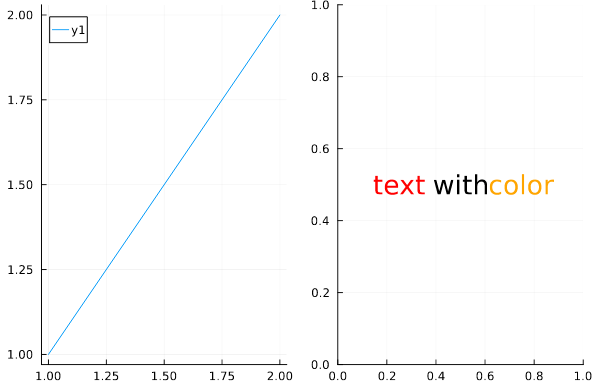# Annotations disappear when put on modified plot

This disscusion presents a way to use annotations to create a multi-colored title, but I have some issues creating annotations on plots that have been modified. Here is a minimal reproducible example using Julia 1.7.2.

``````using Plots
#gr() # The same issue is also present in the gr backend
pyplot()

p = plot()
plot!([1,2],[1,2])
end
tc = [:red, :black, :orange]
for (i,s) in enumerate(["text", "with", "color"])
annotate!(0.25*i , 0.5, text(s, tc[i], 18))
end
return p
end

plot1 = make_plot(, , true)
plot2 = make_plot(, , false)
plot(plot1, plot2)
``````I would have expected the text to appear on top of the line, but instead it is nowhere. Any suggestions?

(PS: If anyone knows a better way to create a multi-colored title using pyplot btw, that would also be welcome.)

There is a problem with the X, Y coordinates in `annotate!()`.
You can add the boolean variable `add_plot` to adjust these in both cases:

``````annotate!(add_plot + 0.25*i , add_plot + 0.5, text(s, tc[i], 18))
``````
1 Like

Thanks! I did try various coordinates in my real plot, but I didn’t know if that was the problem or if there was something else going on, so I had given up. I found them when I tried again now though, so thanks.